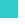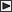BRI Research Paper

 No.83 Flashover Criteria of Compartment Fire -Theory on Zero Order Reaction System-. Y.Hasemi; August, 1979. 26p. Abstract Flashover problem counts undoubtedly among the most important problems in fire safety design, and it is expected that a reasonable and economical design of fire safety in buildings will be performed if a building engineer can estimate the possibility of the occurrence of flashover or predict the time from ignition to flashover in the building or the room that he designs. Many of the studies in the field of fire modeling deal with such problems, and some simulation studies discuss the occurrence of flashover1),2).Though the basic equations of compartment fire involve too many things in comparison with those for the phenomena in engines,heat exchangers or some apparatus, we may succeed in deriving the precise equations of fire whose numerical solution can agree sufficiently with the result of experiment done under the same conditions for the numerical simulation. However, this particular complexity in the formulation of fire takes with another difficulty in applying the equations to the practical problems as mentioned above. In order to make a simulation of fire with these equations, we must prepare a plenty of input data on various parameters in the basic equations as well as initial conditions of variables, but the important problem is that most of them are very capricious and changeable in real world. Thus, numerous calculations and consequently a lot of computer time will be required for the assessment of fire safety even in one compartment. For all these difficulties, we do not need generally so detailed informations on the process of fire, and, from the view point of the design of fire safety, the results obtained with such troubles will come back into only a few categories. The important thing we should do with these equations at first is to clarify what change in the parameters or initial conditions has an important influence on the solution of the basic equations. One of the motives of this study is to get the neccesary informations for fire safety from the basic equations without solving them nor abbreviating the troublesome but important terms out of them. Here we will study the flashover criteria of Control Volume type thermal model, and we have to formulate the flashover at the beginning for this purpose. Though flashover is not clarified sufficiently yet, the temperature-time curve in some zone as shown in Fig.I-1 corresponds undoubtedly to the occurrence of flashover. Temperature does not diverge in an actual fire and converges at. higher temperature, because the oxygen concentration decreases by the rapid oxydization of fuel, but if the solution of the basic equations has a steady state solution in the lower temperature region, flashover as observed in Fig.I-1 does never occur. The flashover problem can be replaced, in such a way, by the problem whether the basic equations have a steady state solution or not, and we will study this problem for the case the reaction follows the 0-order Arrhenius type function. Such an approach was initiated by Semenov3) in the field of thermal explosion theory, but more abstract approach is indispensable for our problem, not only because the equations we deal with involve many knotty terms, but also because the model itself is much more complicated than Semenov's one. Mathematical foundation for some part of this paper depends on the theories of dynamical systems, whose comprehensive introduction is given by Smale and Hirsch4). this paper, we regard that the steady state solution in the physical sense of an ordinary differential equation is expressed mathematically as asymptotically stable equilibrium of the differential equations. Discussion should be made both on the existence of equilibria and on the stability of them. The principal aim of this report is to establish the theoretical basis for the qualitative approach to fire modeling, and the examples studied here are rather simple ones.LinksSitemapInquiryJapanese HP

BUILDING RESEARCH INSTITUTE, JAPAN